# Tutorial 3: ALU Structural Modelling FPGA Implementation

## ALU

ALU internally always do multiple operations like addition, subtraction, division and multiplication. We have to specify which result you want to return as an output by using control lines. In this tutorial, We are implementing 3 bit ALU with Adder, Subtractor, Multiplier and comparator.

To perform this ALU Demo, we are using Slide Switch as Input and LEDs as Output on EDGE Spartan 6 FPGA Kit.Each of the Modules are constructed structurally for better understanding of combination circuit design and port mapped to the Top level ALU.

For behavioural implementation of ALU refer the VHDL code for 4 bit ALU .

## Block diagram of the ALU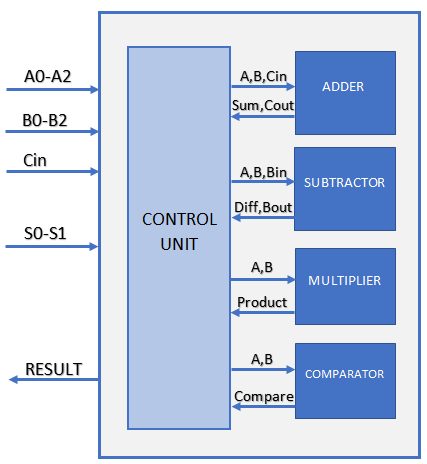Top Module consist of 3 bit Adder, subractor, multiplier and comparator as a Port mapped components and 2 bit mux to select the output result.

## VHDL Code for Top Module ALU

```
library IEEE;
use IEEE.STD_LOGIC_1164.ALL;

entity ALU is
Port ( a : in STD_LOGIC_VECTOR (2 downto 0);
b : in STD_LOGIC_VECTOR (2 downto 0);
c: in std_logic;
sel : in STD_LOGIC_VECTOR (1 downto 0);
result : out STD_LOGIC_VECTOR (5 downto 0));
end ALU;

architecture Behavioral of ALU is

component comparator_3bit
port( a : in std_logic_vector(2 downto 0);
b : in std_logic_vector(2 downto 0);
less : out std_logic;
equal : out std_logic;
greater : out std_logic);
end component;

component multiplier_3bit
Port ( a : in STD_LOGIC_VECTOR (2 downto 0);
b : in STD_LOGIC_VECTOR (2 downto 0);
p : out STD_LOGIC_VECTOR (5 downto 0));
end component;

component subtractor_3bit
Port ( a : in STD_LOGIC_VECTOR (2 downto 0);
b : in STD_LOGIC_VECTOR (2 downto 0);
bin : in STD_LOGIC;
diff : out STD_LOGIC_VECTOR (2 downto 0);
bout : out STD_LOGIC);
end component;

Port ( a : in STD_LOGIC_VECTOR (2 downto 0);
b : in STD_LOGIC_VECTOR (2 downto 0);
cin : in STD_LOGIC;
sum : out STD_LOGIC_VECTOR (2 downto 0);
cout : out STD_LOGIC);
end component;

signal t_comp,t_multi,t_sub,t_adder:std_logic_vector(5 downto 0):= (others => '0');

begin

alu2: subtractor_3bit port map(a=>a,b=>b,bin=>c,diff=>t_sub(2 downto 0),bout=>t_sub(3));
alu3: multiplier_3bit port map(a=>a,b=>b,p=>t_multi);
alu4: comparator_3bit port map(a=>a,b=>b,less=>t_comp(0),equal=>t_comp(1),greater=>t_comp(2));

begin
case sel is
when "00"=>
result<=t_sub; when "10"=>
result<=t_multi; when "11"=>
result<=t_comp; when others =>
result<="000000";
end case;
end process;

end Behavioral;

```

Schematic diagram shows the complete ALU architecture implemented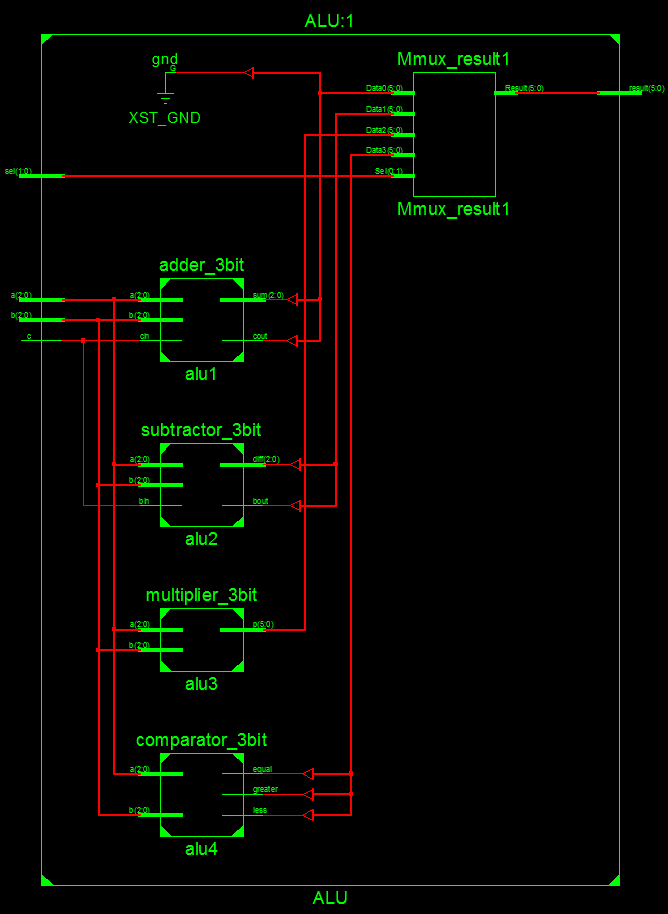3 bit Adder unit is constructed using Full Adder. Full Adder is constructed using Half Adder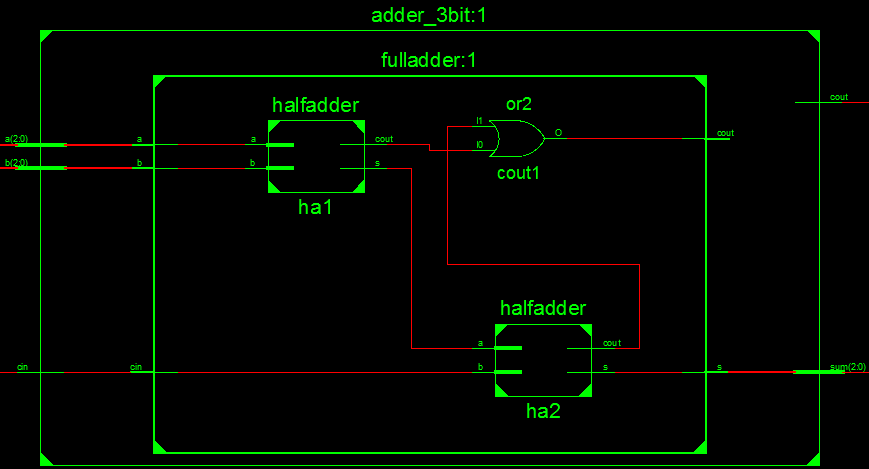## VHDL Code for 3 bit Adder

```
library IEEE;
use IEEE.STD_LOGIC_1164.ALL;

Port ( a : in STD_LOGIC_VECTOR (2 downto 0);
b : in STD_LOGIC_VECTOR (2 downto 0);
cin : in STD_LOGIC;
sum : out STD_LOGIC_VECTOR (2 downto 0);
cout : out STD_LOGIC);

architecture Behavioral of adder_3bit is

port( a : in STD_LOGIC;
b : in STD_LOGIC;
cin : in STD_LOGIC;
s : out STD_LOGIC;
cout : out STD_LOGIC);
end component;

signal c1,c2:std_logic;

begin

end Behavioral;

```

## VHDL Code for Full Adder

```
library IEEE;
use IEEE.STD_LOGIC_1164.ALL;

port( a : in STD_LOGIC;
b : in STD_LOGIC;
cin : in STD_LOGIC;
s : out STD_LOGIC;
cout : out STD_LOGIC);

architecture Behavioral of fulladder is

Port( a,b : in std_logic;
s : out STD_LOGIC;
cout : out STD_LOGIC);
end component;

signal s1,c1,c2:std_logic;

begin

ha1: halfadder port map(a,b,s1,c1);
ha2: halfadder port map(s1,cin,s,c2);
cout <= c1 or c2;

end Behavioral;

```

## VHDL Code for Half Adder

```
library IEEE;
use IEEE.STD_LOGIC_1164.ALL;

Port( a,b : in std_logic;
s : out STD_LOGIC;
cout : out STD_LOGIC);

architecture Behavioral of halfadder is

begin

s <= a xor b;
cout <= a and b;

end Behavioral;

```

3 Bit subtractor is constructed using 3 full subtractors as shown in schematics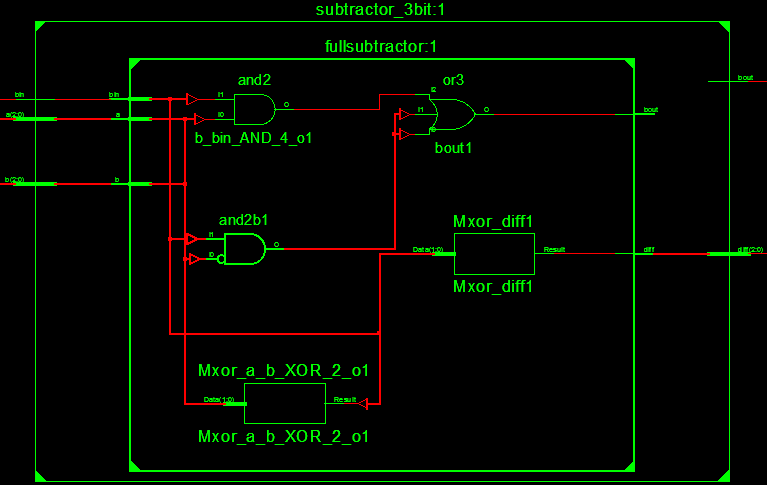## VHDL Code for 3 bit Subtractor

```
library IEEE;
use IEEE.STD_LOGIC_1164.ALL;

entity subtractor_3bit is
Port ( a : in STD_LOGIC_VECTOR (2 downto 0);
b : in STD_LOGIC_VECTOR (2 downto 0);
bin : in STD_LOGIC;
diff : out STD_LOGIC_VECTOR (2 downto 0);
bout : out STD_LOGIC);
end subtractor_3bit;

architecture Behavioral of subtractor_3bit is

component fullsubtractor is
Port( a : in STD_LOGIC;
b : in STD_LOGIC;
bin : in STD_LOGIC;
diff : out STD_LOGIC;
bout : out STD_LOGIC);
end component;

signal br0,br1: std_logic;

begin

fs1: fullsubtractor port map(a(0),b(0),bin,diff(0),br0);
fs2: fullsubtractor port map(a(1),b(1),br0,diff(1),br1);
fs3: fullsubtractor port map(a(2),b(2),br1,diff(2),bout);

end Behavioral;

```

## VHDL Code for Full Subtractor

```
library IEEE;
use IEEE.STD_LOGIC_1164.ALL;

entity fullsubtractor is
Port ( a : in STD_LOGIC;
b : in STD_LOGIC;
bin : in STD_LOGIC;
diff : out STD_LOGIC;
bout : out STD_LOGIC);
end fullsubtractor;

architecture Behavioral of fullsubtractor is

begin

diff <= a xor b xor bin;
bout <= ((not a)and b)or((not a)and bin)or(b and bin);

end Behavioral;

```

3 Bit multiplier is implemented using Full Adder, Half Adder and AND gates as shown in Schematics## VHDL Code for 3 bit Multiplier

```library IEEE;
use IEEE.STD_LOGIC_1164.ALL;

entity multiplier_3bit is
Port( a : in STD_LOGIC_VECTOR (2 downto 0);
b : in STD_LOGIC_VECTOR (2 downto 0);
p : out STD_LOGIC_VECTOR (5 downto 0));
end multiplier_3bit;

architecture Behavioral of multiplier_3bit is

Port( a : in STD_LOGIC;
b : in STD_LOGIC;
cin : in STD_LOGIC;
s : out STD_LOGIC;
cout : out STD_LOGIC);
end component;

port( a,b : in std_logic;
s : out STD_LOGIC;
cout : out STD_LOGIC);
end component;

signal f0,f1,f2,f3,f4,f5,f6,f7,f8,f9,f10,f11,f12,s1,s2: std_logic;

begin

ha1: halfadder port map(f0,f1,p(1),f2);
fa1: fulladder port map(f2,f3,f4,s1,f5);
ha2: halfadder port map (f5,f6,s2,f7);
ha3: halfadder port map (s1,f8,p(2),f9);
fa2: fulladder port map (f9,s2,f10,p(3),f11);
fa3: fulladder port map (f11,f7,f12,p(4),p(5));

f0 <= a(0) and b(1);
f1 <= a(1) and b(0);
f3 <= a(0) and b(2);
f4 <= a(1) and b(1);
f6 <= a(1) and b(2);
f8 <= a(2) and b(0);
f10<= a(2) and b(1);
f12<= a(2) and b(2);
P(0)<=a(0) and b(0);

end Behavioral;```

Finally comparator unit is constructed behaviourally as shown in schematics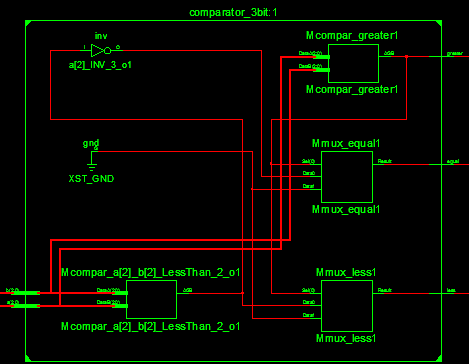## VHDL Code for 3 bit comparator

```library IEEE;
use IEEE.STD_LOGIC_1164.ALL;

entity comparator_3bit is
Port ( a : in std_logic_vector(2 downto 0);
b : in std_logic_vector(2 downto 0);
less : out std_logic;
equal : out std_logic;
greater : out std_logic);
end comparator_3bit;

architecture Behavioral of comparator_3bit is

begin

process(a,b)
begin
if (a > b ) then --condition a is greater than b
less <= '0';
equal <= '0';
greater <= '1';
elsif (a < b) then --condition a is less than b
less <= '1';
equal <= '0';
greater <= '0';
else --condition a is equals b
less <= '0';
equal <= '1';
greater <= '0';
end if;
end process;

end Behavioral;```

## Pin Assignment

Lets assign the User Constraint file of EDGE Spartan 6 FPGA kit to ALU

Input A0, A1, A2 ar assigned to Slide switches from LSB.

```NET "a(0)" LOC="P22";
NET "a(1)" LOC="P21";
NET "a(2)" LOC="P17"; ```

Input B0,B1,B2 are assigned slide switches next to A input

```NET "b(0)" LOC="P16";
NET "b(1)" LOC="P15";
NET "b(2)" LOC="P14";```

Carry input is assigned next switch of B input

`NET "c" LOC="P12"; `

Selection lines S0 and S1 are assigned to Slide switches at the MSB Position

```NET "sel(0)" LOC="P2";
NET "sel(1)" LOC="P1"; ```

Results 6bit output are assigned to LEDs from LSB position.

```NET "result(0)" LOC="P33";
NET "result(1)" LOC="P32";
NET "result(2)" LOC="P30";
NET "result(3)" LOC="P29";
NET "result(4)" LOC="P27";
NET "result(5)" LOC="P26";```